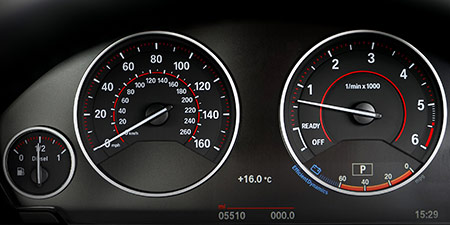# Kilometers per hour (km/h) to Knot (kn)

Units of speed ﹣ Converter Kilometers per hour to Knot

Here you can convert the Speed unit Kilometers per hour into the unit Knot and vice versa you can convert Knot into Kilometers per hour. By clicking the "Swap units" icon, you will always obtain the desired conversion in the calculation result, i.e., km/h to kn or kn to km/h. With the following calculator you can also calculate any other Speed unit.

## Info about "Kilometers per hour"

Kilometers per hour is used as a standard unit in transportation, therefore it is used in traffic or to measure the travel speed of vehicles. For example, a commercial aircraft flies at a speed of 1,000 km/h then it covers a distance of 1,000 kilometers per hour.

The unit is defined as follows: An object moving at 1 "km/h" per hour will cover one kilometer. "km/h" is approved to use in the international system of units (SI).

Definition: An object that moves with a knot for one hour will cover a distance of one nautical mile. Therefore 1 nautical mile is equivalent to 1,852 meters. A knot refers to the speed of one nautical mile per hour.

The following rule is an easy way to remember when converting knots into km/h: "Multiply by two, and minus 10%". For example, a ship travelling at 20 knots will have a speed around 40-4=36 km/h (exactly 37.04 km/h).

## Basis for conversion Kilometers per hour (km/h) to Knot (kn) and vice versa

The abbreviation for the "Speed unit Kilometers per hour" is km/h. The abbreviation for the "Speed unit Knot" is kn.

## Formula for the conversion of Kilometers per hour (km/h) to Knot (kn) and vice versa

The calculation from Kilometers per hour to Knot shall be made using the following conversion formula:

Conversion formula Kilometers per hour to Knot

Determine the number of Knot from Kilometers per hour

Kilometers per hour × 0.53995680345572

## Formula for the conversion of Knot (kn) to Kilometers per hour (km/h)

The calculation from Knot to Kilometers per hour shall be made using the following conversion formula:

Conversion formula Knot to Kilometers per hour

Determine the number of Kilometers per hour from Knot

Knot × 1.852

## Overview table : How many Kilometers per hour are in a Knot ?

Kilometers per hour km/h ⇒ Knot kn
0.01 km/h  are  0.00540 kn
0.02 km/h  are  0.01079 kn
0.03 km/h  are  0.01619 kn
0.04 km/h  are  0.02159 kn
0.05 km/h  are  0.02699 kn
0.06 km/h  are  0.03239 kn
0.07 km/h  are  0.03779 kn
0.08 km/h  are  0.04319 kn
0.09 km/h  are  0.04859 kn
0.10 km/h  are  0.05399 kn
0.20 km/h  are  0.10799 kn
0.30 km/h  are  0.16198 kn
0.40 km/h  are  0.21598 kn
0.50 km/h  are  0.26997 kn
0.60 km/h  are  0.32397 kn
0.70 km/h  are  0.37797 kn
0.80 km/h  are  0.43196 kn
0.90 km/h  are  0.48596 kn
1 km/h  corresponds to  0.53995 kn
2 km/h  are  1.07991 kn
3 km/h  are  1.61987 kn
4 km/h  are  2.15982 kn
5 km/h  are  2.69978 kn
6 km/h  are  3.23974 kn
7 km/h  are  3.77969 kn
8 km/h  are  4.31965 kn
9 km/h  are  4.85961 kn
10 km/h  are  5.39956 kn
20 km/h  are  10.79913 kn
30 km/h  are  16.19870 kn
40 km/h  are  21.59827 kn
50 km/h  are  26.99784 kn
60 km/h  are  32.39740 kn
70 km/h  are  37.79697 kn
80 km/h  are  43.19654 kn
90 km/h  are  48.59611 kn
100 km/h  are  53.99568 kn
200 km/h  are  107.99136 kn
300 km/h  are  161.98704 kn
400 km/h  are  215.98272 kn
500 km/h  are  269.97840 kn
600 km/h  are  323.97408 kn
700 km/h  are  377.96976 kn
800 km/h  are  431.96544 kn
900 km/h  are  485.96112 kn
1 000 km/h  are  539.95680 kn

## Overview table : How many Knot are in a Kilometers per hour ?

Knot kn ⇒ Kilometers per hour km/h
0.01 kn  are  0.01852 km/h
0.02 kn  are  0.03704 km/h
0.03 kn  are  0.05556 km/h
0.04 kn  are  0.07408 km/h
0.05 kn  are  0.09260 km/h
0.06 kn  are  0.11112 km/h
0.07 kn  are  0.12964 km/h
0.08 kn  are  0.14816 km/h
0.09 kn  are  0.16668 km/h
0.10 kn  are  0.18520 km/h
0.20 kn  are  0.37040 km/h
0.30 kn  are  0.55560 km/h
0.40 kn  are  0.74080 km/h
0.50 kn  are  0.92600 km/h
0.60 kn  are  1.11120 km/h
0.70 kn  are  1.29640 km/h
0.80 kn  are  1.48160 km/h
0.90 kn  are  1.66680 km/h
1 kn  corresponds to  1.85200 km/h
2 kn  are  3.70400 km/h
3 kn  are  5.55600 km/h
4 kn  are  7.40800 km/h
5 kn  are  9.26000 km/h
6 kn  are  11.11200 km/h
7 kn  are  12.96400 km/h
8 kn  are  14.81600 km/h
9 kn  are  16.66800 km/h
10 kn  are  18.52000 km/h
20 kn  are  37.04000 km/h
30 kn  are  55.56000 km/h
40 kn  are  74.08000 km/h
50 kn  are  92.60000 km/h
60 kn  are  111.12000 km/h
70 kn  are  129.64000 km/h
80 kn  are  148.16000 km/h
90 kn  are  166.68000 km/h
100 kn  are  185.20000 km/h
200 kn  are  370.40000 km/h
300 kn  are  555.60000 km/h
400 kn  are  740.80000 km/h
500 kn  are  926 km/h
600 kn  are  1 111.20000 km/h
700 kn  are  1 296.40000 km/h
800 kn  are  1 481.60000 km/h
900 kn  are  1 666.80000 km/h
1 000 kn  are  1 852 km/h

## Source information

As source for the information in the 'Units of speed' category, we have used in particular:

## Last update on February 19, 2023

The pages of the 'Units of speed' category were last editorially reviewed by Stefan Banse on February 19, 2023. They all correspond to the current status.

### Previous changes on October 9, 2021

• November 9, 2020: Publication of the Speed converter
• Editorial revision of all texts in this category